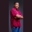Related Tags

swift
communitycreator

# Fetching the last element to satisfy a condition in Swift arraysTheodore Kelechukwu Onyejiaku

### Overview

We use the last(where:) method to get the last element of an array that satisfies a given condition.

### Syntax

arr.last(where: condition)


### Parameters

arr: This is the array for which we want to find the last element, in order to satisfy a given condition.

Condition: This closure takes an array element as its argument and returns a Boolean value, indicating whether the element matches the condition.

### Return value

What is returned is the last element of the array that satisfies the condition. Otherwise, nothing is returned if there is no element that satisfies the condition.

### Code

let evenNumbers = [2, 4, 6, 8, 10]
let twoLetterWords = ["up", "go", "me", "we"]
let numbers = [2, 3, -4, 5, -9]

// invoke the last(where:) method
let lastGreaterThanFive = evenNumbers.last(where: {$0 >= 5})! let lastTwoLetters = twoLetterWords.last(where: {$0.count == 2})!
let lastNegative = numbers.last(where: {\$0 < 0})!

// print results
print(lastGreaterThanFive)
print(lastTwoLetters)
print(lastNegative)
Getting the last element of an array that satisfies a condition

### Explanation

• Lines 1-3: We create three arrays.
• Lines 6-8: We invoke the last(where:) method on the arrays we created and store the returned results in some variables.
• Lines 11-13: We print the results.

RELATED TAGS

swift
communitycreator

CONTRIBUTORTheodore Kelechukwu Onyejiaku
RELATED COURSES

View all Courses

Keep Exploring

Learn in-demand tech skills in half the time